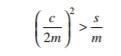LATEST UPDATES

## Friday, 11 November 2016

### Frequency of Free Damped Vibrations (Viscous Damping)

We have already know that the motion of a body is resisted by frictional forces. In vibrating systems, the effect of friction is referred to as "Damping". The Damping provided by fluid resistance is known as viscous damping.
We have also discussed that in damped vibrations, the amplitude of the resulting vibration gradually diminishes. This is due to the reason that a certain amount of energy is always dissipated to overcome the frictional resistance. The resistance to the motion of the body is provided partly by the medium in which the vibration takes place and partly by the internal friction, and in some cases partly by a dash pot or other external damping device.
Consider a vibrating system, as shown in Fig., in which a mass is suspended from one end of the spiral spring and the other end of which is fixed. A damper is provided between the mass and the rigid support.

Let          m = Mass suspended from the spring,
s = Stiffness of the spring,
x = Displacement of the mass from the mean position at time t,
δ = Static deflection of the spring
= m.g/s, and
c = Damping coefficient or the damping force per unit velocity.

Since in viscous damping, it is assumed that the frictional resistance to the motion of the body is directly proportional to the speed of the movement, therefore
Damping force or frictional force on the mass acting in opposite direction to the motion of the mass
= c . dx/dt
Accelerating force on the mass, acting along the motion of the mass
= m . d2 x/dt2
and spring force on the mass. acting in opposite direction to the motion of the mass,
= s.x
Therefore the equation of motion becomes
This is differential equation of second order. Assuming a solution of the form x = ekt , where 'k' is a constant to be determined. Now the above differential equation reduces to
The two roots of the equation are
The most general solution of the differential solution (i) with its right hand side equal to zero has only complementary function and it is given by
Where Cand  Care two arbitrary constants which are to be determined from the initial conditions of the motion of the mass.
It may be noted that the roots Kand K2 may be real, complex conjugate (imaginary) or equal. We shall now discuss the three cases as below:

Case 1: When the roots are real (overdamping)
If ,, then the roots Kand Kare equal and negative. This is a case of Over damping or Large damping and the mass moves slowly to the equilibrium position. This motion is known as aperiodic, when the roots are real, the most general solution of the differential equation is

Note: In actual practice, the overdamped vibrations are avoided.

Case  2: When the roots are complex conjugate (underdamping)
The two roots K1  and Kare then known as complex conjugate. This is a most practical case of damping and it is known as Under damping or Small damping. the roots are
We see from equation (iv), that the motion of the mass is simple harmonic whose circular damped frequency is  ωd and the amplitude ae-at  which diminishes exponentially with time as shown in Fig., Though the mass eventually returns to its equilibrium position because of its inertia, yet it overshoots and the oscillations may take some considerable time to die away.

Note: When no damper is provided in the system, then C = 0. Therefore the frequency of the undamped vibration,
It is the same as discussed under free-vibrations.

Case 3. When the roots are equal (critical damping)then the radical (i.e., the term under the square root) becomes zero and the two roots K and Kare equal. This is a case of Critical damping. In other words, the critical damping is said to occur when frequency of damped vibration ( fd) is zero (i.e motion is aperiodic). This type of damping is also avoided because the mass moves back rapidly to its equilibrium position, in the shortest possible time.
For critical damping, equation (ii) may be written as
Thus the motion is again aperiodic. The critical damping coefficient (CC) may be obtained by substituting Cfor C in the condition for critical damping, i.e.,
The critical damping coefficient is the amount of damping required for a system to be critically damped.

#### Post a Comment

@2017 All Rights Reserved. Designed by WWW.SMARTWAY4STUDY.COM !!!! Sitemap !!!! Blogger Templates